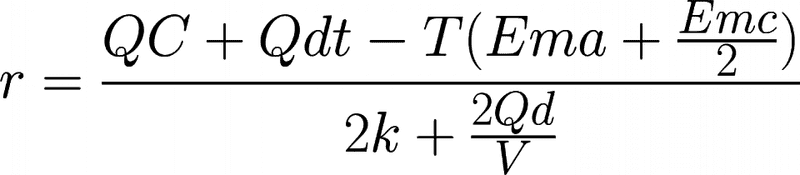# How to calculate the foraging radius of a bird?

Manassa Mauler
I would love someone to verify the answer for equation 8 in this paper (bottom of page 263) http://onlinelibrary.wiley.com/doi/10.1046/j.1365-2028.2002.00368.x/epdf

For the sake of clarity here is the equation is LaTeX which you can render at the following link
\frac{QC + Q\lambda \sigma - T(_{Ema + \frac{_{Emc}}{2}}))}{2k + \frac{2Qd}{V}}
https://www.codecogs.com/latex/eqneditor.php

The inputs are:
Q = 5.2 * 10^6 Joules
C =1.5 kg
lambda = 0.055 kg per hour
sigma = 12 hours
T = 48 hours
Ema = 42 Watts
Emc =24 Watts
k=2 Joules per meter
V =45 km per hour
The answer should be 205 km

Thanks!

Last edited:

Mentor
$\frac{QC + Q\lambda \sigma - T(_{Ema + \frac{_{Emc}}{2}}))}{2k + \frac{2Qd}{V}}$

Mentor
Well. it seems to me the parentheses are unbalanced in the numerator, is one "open" parenthesis missing somewhere? Or can we assume it is okay to drop the very last one?

Manassa Mauler
I apologise but I was incorrect when I looked at it again. Here is the correct version
Q <- 5.2 * 10^6 Joules
C <- 1.5 kg
d <- 0.055 kg per hour
t <- 12 hours
Time <- 48 hours
Ema <- 24 Watts
Emc <- 42 Watts
k <- 2 joules per meter
V <- 45 km per hour
Thanks for looking at this JimMentor
Show your work, please. I get 1693. But what you posted may not be what you and I solved.
My calculation seems ridiculous to me. So I am guessing the formula has a problem - or I can't do arithmetic. Either is possible. Plus, I think there may be a problem with units.

PS: the paper you linked is behind a paywall for me. So I cannot verify anything including my work.

Last edited: